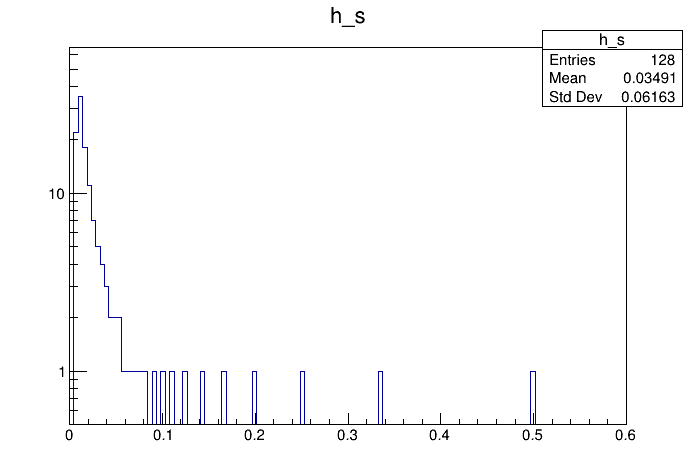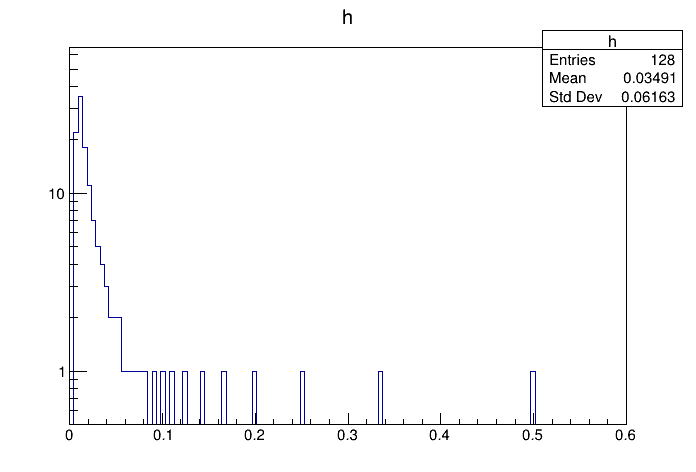# Df 0 1 0_Trivial Data Source¶

Use the "trivial data source", an example data source implementation.

This tutorial illustrates how use the RDataFrame in combination with a RDataSource. In this case we use a RTrivialDS, which is nothing more than a simple generator: it does not interface to any existing dataset. The RTrivialDS has a single column, col0, which has value n for entry n. The code for RTrivialDS is available at these links (header and source):

Author: Danilo Piparo (CERN)
This notebook tutorial was automatically generated with ROOTBOOK-izer from the macro found in the ROOT repository on Thursday, June 24, 2021 at 07:13 AM.

In :
auto nEvents = 128U;
auto d_s = ROOT::RDF::MakeTrivialDataFrame(nEvents);


/ now we have a regular rdataframe: the ingestion of data is delegated to / the RDataSource. At this point everything works as before.

In :
auto h_s = d_s.Define("x", "1./(1. + col0)").Histo1D({"h_s", "h_s", 128, 0, .6}, "x");


/ now we redo the same with a rdf from scratch and we draw the two histograms

In :
ROOT::RDataFrame d(nEvents);


/ this lambda redoes what the ttrivialds provides

In :
auto g = []() {
static ULong64_t i = 0;
return i++;
};
auto h = d.Define("col0", g).Define("x", "1./(1. + col0)").Histo1D({"h", "h", 128, 0, .6}, "x");

auto c_s = new TCanvas();
c_s->SetLogy();
h_s->DrawClone();

auto c = new TCanvas();
c->SetLogy();
h->DrawClone();

return 0;


Draw all canvases

In :
gROOT->GetListOfCanvases()->Draw()